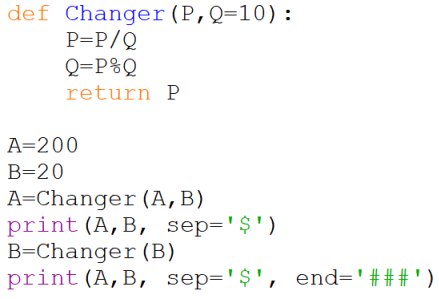CBSE Class 12 Sample Paper for 2024 Boards

Computer Science - Class 12
Solutions to CBSE Sample Paper - Computer Science Class 12

##googletag.cmd.push(function() { googletag.display('div-gpt-ad-1669298377854-0'); });

The output of the code is:

10.0\$20

10.0\$2.0###

• The code defines a function named Changer that takes two parameters P and Q , where Q has a default value of 10 . The function performs the following operations:
• It divides P by Q and assigns the result to P .
• It returns the remainder of P divided by Q and assigns it to Q .
• It returns P from the function.
• The code assigns 200 to A and 20 to B , and then calls the Changer function with A and B as arguments. The function returns 10.0 and assigns it to A. Then it prints A and B separated by a dollar sign (\$), which is 10.0\$20.
• The code calls the Changer function with B as the only argument. The function takes 10 as the default value for Q, and returns 10.0 and assigns it to B. Then it prints A and B separated by a dollar sign (\$) followed by three hashes (###), which is 10.0\$2.0###.

The reason why the output has decimal values is because of the type conversion that happens when dividing two integers in Python. In Python 3, the division operator (/) always returns a float value, even if the operands are integers. For example, 200/20 returns 10.0, not 10. A float value is a decimal number that can have fractional parts, such as 10.0, 0.5, 3.14, etc.

10.0\$20

10.0\$2.0###

Learn in your speed, with individual attention - Teachoo Maths 1-on-1 Class